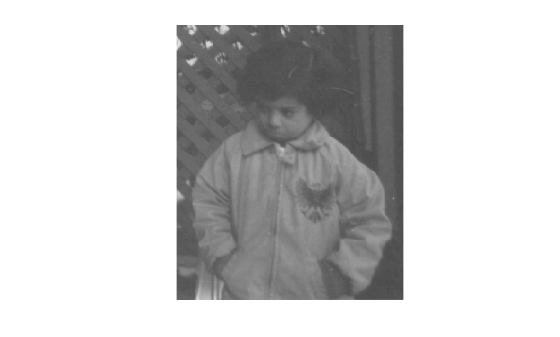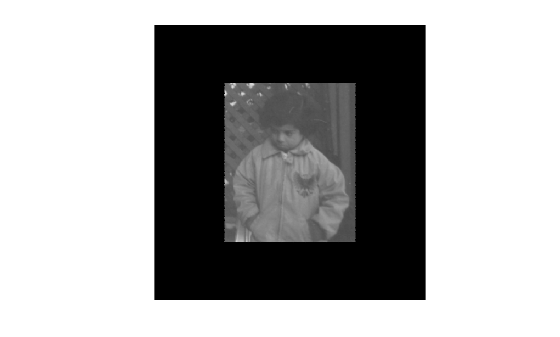invert

Invert geometric transformation

Description

example

invtform = invert(tform) returns the inverse of the geometric transformation tform.

Examples

collapse all

imshow(I)Create an affine2d object that defines a 30 degree clockwise rotation around the origin. View the transformation matrix stored in the T property.

theta = 30;
tform = affine2d([cosd(theta) sind(theta) 0; -sind(theta) cosd(theta) 0; 0 0 1]);
tform.T
ans = 3×3

0.8660    0.5000         0
-0.5000    0.8660         0
0         0    1.0000

Apply the forward geometric transformation, tform, to the image. Display the rotated image.

J = imwarp(I,tform);
imshow(J)Invert the geometric transformation. The result is a new affine2d object that represents a 30 degree rotation in the counterclockwise direction.

invtform = invert(tform);
invtform.T
ans = 3×3

0.8660   -0.5000         0
0.5000    0.8660         0
0         0    1.0000

Apply the inverse geometric transformation, invtform, to the rotated image J. The final image, K, has the same size, shape, and orientation as the original image. Black padding around the image results from the two transformations.

K = imwarp(J,invtform);
imshow(K)Input Arguments

collapse all

Geometric transformation, specified as an affine2d, affine3d, rigid2d, rigid3d, or projective2d geometric transformation object.

Output Arguments

collapse all

Inverse geometric transformation, returned as a geometric transformation object. invtform is the same type of object as tform.